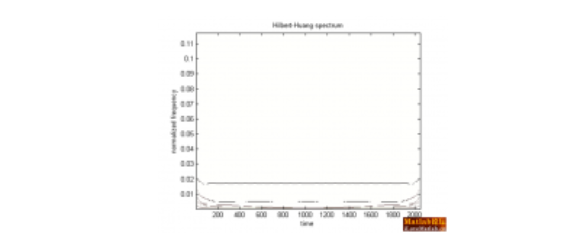function HHT
clear;clc;clf;
N=2048;
%fft默认计算的信号是从0开始的
t=linspace(1,2,N);deta=t(2)-t(1);fs=1/deta;
x=5*sin(2*pi*10*t)+5*sin(2*pi*35*t);
z=x;
c=emd(z);

%计算每个IMF分量及最后一个剩余分量residual与原始信号的相关性
[m,n]=size(c);
for i=1:m;
a=corrcoef(c(i,:),z);
xg(i)=a(1,2);
end
xg;

for i=1:m-1
%--------------------------------------------------------------------
%计算各IMF的方差贡献率
%定义：方差为平方的均值减去均值的平方
%均值的平方
%imfp2=mean(c(i,:),2).^2
%平方的均值
%imf2p=mean(c(i,:).^2,2)
%各个IMF的方差
mse(i)=mean(c(i,:).^2,2)-mean(c(i,:),2).^2;
end;
mmse=sum(mse);
for i=1:m-1
mse(i)=mean(c(i,:).^2,2)-mean(c(i,:),2).^2;
%方差百分比，也就是方差贡献率
mseb(i)=mse(i)/mmse*100;
%显示各个IMF的方差和贡献率
end;
%画出每个IMF分量及最后一个剩余分量residual的图形
figure(1)
for i=1:m-1
disp(['imf',int2str(i)]) ;disp([mse(i) mseb(i)]);
end;
subplot(m+1,1,1)
plot(t,z)
set(gca,'fontname','times New Roman')
set(gca,'fontsize',14.0)
ylabel(['signal','Amplitude'])

for i=1:m-1
subplot(m+1,1,i+1);
set(gcf,'color','w')
plot(t,c(i,:),'k')
set(gca,'fontname','times New Roman')
set(gca,'fontsize',14.0)
ylabel(['imf',int2str(i)])
end
subplot(m+1,1,m+1);
set(gcf,'color','w')
plot(t,c(m,:),'k')
set(gca,'fontname','times New Roman')
set(gca,'fontsize',14.0)
ylabel(['r',int2str(m-1)])

%画出每个IMF分量及剩余分量residual的幅频曲线
figure(2)
subplot(m+1,1,1)
set(gcf,'color','w')
[f,z]=fftfenxi(t,z);
plot(f,z,'k')
set(gca,'fontname','times New Roman')
set(gca,'fontsize',14.0)
ylabel(['initial signal',int2str(m-1),'Amplitude'])

for i=1:m-1
subplot(m+1,1,i+1);
set(gcf,'color','w')
[f,z]=fftfenxi(t,c(i,:));
plot(f,z,'k')
set(gca,'fontname','times New Roman')
set(gca,'fontsize',14.0)
ylabel(['imf',int2str(i),'Amplitude'])
end
subplot(m+1,1,m+1);
set(gcf,'color','w')
[f,z]=fftfenxi(t,c(m,:));
plot(f,z,'k')
set(gca,'fontname','times New Roman')
set(gca,'fontsize',14.0)
ylabel(['r',int2str(m-1),'Amplitude'])

hx=hilbert(z);
xr=real(hx);xi=imag(hx);
%计算瞬时振幅
sz=sqrt(xr.^2+xi.^2);
%计算瞬时相位
sx=angle(hx);
%计算瞬时频率
dt=diff(t);
dx=diff(sx);
sp=dx./dt;
figure(6)
plot(t(1:N-1),sp)
title('瞬时频率')

%计算HHT时频谱和边际谱
[A,fa,tt]=hhspectrum(c);
[E,tt1]=toimage(A,fa,tt,length(tt));
figure(3)
disp_hhs(E,tt1) %二维图显示HHT时频谱，E是求得的HHT谱
pause
figure(4)
for i=1:size(c,1)
faa=fa(i,:);
[FA,TT1]=meshgrid(faa,tt1);%三维图显示HHT时频图
surf(FA,TT1,E)
title('HHT时频谱三维显示')
hold on
end
hold off
E=flipud(E);
for k=1:size(E,1)
bjp(k)=sum(E(k,:))*1/fs;
end
f=(1:N-2)/N*(fs/2);
figure(5)
plot(f,bjp);
xlabel('频率 / Hz');
ylabel('信号幅值');
title('信号边际谱')%要求边际谱必须先对信号进行EMD分解

function [A,f,tt] = hhspectrum(x,t,l,aff)

error(nargchk(1,4,nargin));

if nargin < 2

t=1:size(x,2);

end

if nargin < 3

l=1;

end

if nargin < 4

aff = 0;

end

if min(size(x)) == 1
if size(x,2) == 1
x = x';
if nargin < 2
t = 1:size(x,2);
end
end
Nmodes = 1;
else
Nmodes = size(x,1);
end

lt=length(t);

tt=t((l+1):(lt-l));

for i=1:Nmodes

an(i,:)=hilbert(x(i,:)')';
f(i,:)=instfreq(an(i,:)',tt,l)';
A=abs(an(:,l+1:end-l));

if aff
disprog(i,Nmodes,max(Nmodes,100))
end

end

function disp_hhs(im,t,inf)

% DISP_HHS(im,t,inf)
% displays in a new figure the spectrum contained in matrix "im"
% (amplitudes in log).
%
% inputs : - im : image matrix (e.g., output of "toimage")
% - t (optional) : time instants (e.g., output of "toimage")
% - inf (optional) : -dynamic range in dB (wrt max)
% default : inf = -20
%
% utilisation : disp_hhs(im) ; disp_hhs(im,t) ; disp_hhs(im,inf)
% disp_hhs(im,t,inf)

figure
colormap(bone)
colormap(1-colormap);

if nargin==1
inf=-20;
t = 1:size(im,2);

end

if nargin == 2
if length(t) == 1
inf = t;
t = 1:size(im,2);
else
inf = -20;
end
end

if inf >= 0
error('inf doit etre < 0')
end

M=max(max(im));

im = log10(im/M+1e-300);

inf=inf/10;

imagesc(t,fliplr((1:size(im,1))/(2*size(im,1))),im,[inf,0]);
set(gca,'YDir','normal')
xlabel(['time'])
ylabel(['normalized frequency'])
title('Hilbert-Huang spectrum')
function [f,z]=fftfenxi(t,y)
L=length(t);N=2^nextpow2(L);
%fft默认计算的信号是从0开始的
t=linspace(t(1),t(L),N);deta=t(2)-t(1);
m=0:N-1;
f=1./(N*deta)*m;
%下面计算的Y就是x(t)的傅里叶变换数值
%Y=exp(i*4*pi*f).*fft(y)%将计算出来的频谱乘以exp(i*4*pi*f)得到频移后[-2,2]之间的频谱值
Y=fft(y);
z=sqrt(Y.*conj(Y));1个回答